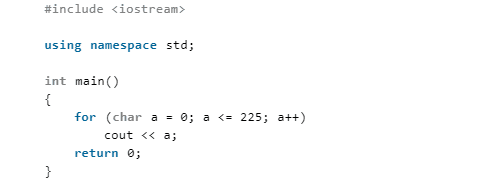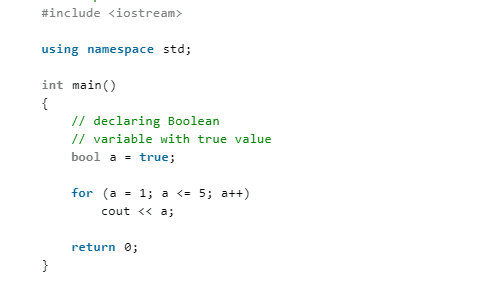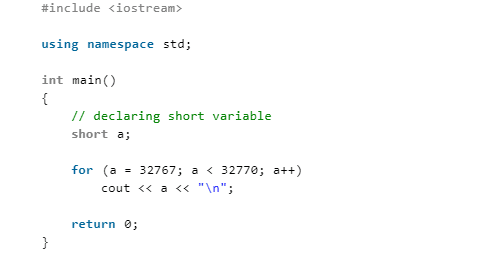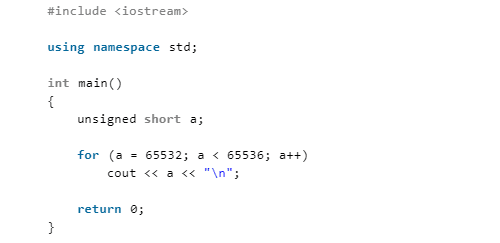# Notes on C / C + + Programming: what should we do when the C + + data type exceeds the valid range?

Time：2021-6-16

First, let’s consider the following procedure.

1) The program shows what happens when we cross the ‘char’ range:Will this code print “a” until it becomes 226? The answer is an indefinite loop, because the “a” here is declared as a character and its valid range is – 128 to + 127. When “a” is changed to 128 by a + +, it is out of range. As a result, the first number (i.e. – 128) starting from the negative number of the range is assigned to a. Therefore, the condition “a < = 225” is satisfied and the control remains in the loop.

2) The program shows what happens when we cross the ‘bool’ range:The code will print “1” indefinitely because “a” here is declared as “bool” and the valid range is 0 to 1. For Boolean variables, everything except 0 is 1 (or true). When “a” attempts to become 2 (via a + +), 1 is assigned to “a”. The condition a < = 5 is satisfied and the control remains in the loop.

3) The program shows what happens when we cross the ‘short’ range:

Note that short is short for short int. They are synonyms. Short, short int, signed short and signed short int are the same data types.Will this code print ‘a’ until it becomes 32770? The answer is an indefinite loop, because the “a” here is declared as short, and its valid range is – 32768 to + 32767. When “a” tries to change to 32768 through a + +, it is out of range. As a result, the first digit of the negative number in the range (i.e. – 32768) is assigned to a. Therefore, the condition “a < 32770” is satisfied and the control is still in the cycle.

4) The program shows what happens when we cross the ‘unsigned short’ range:Will this code print “a” until it becomes 65536? The answer is an indefinite loop, because the “a” here is declared short, and its valid range is 0 to + 65535. When “a” tries to change to 65536 through a + +, it is out of range, and the result is that the first number (0) in the range is assigned to a. Therefore, the condition “a < 65536” is satisfied and the control is still in the cycle.

Explanation-

We know that computers use the complement of 2 to represent data. For example, if we have a byte (we can look at it in decimal form with char and% d as the format specifier), we can say – 128 to 127. If we add 127 to 1, we get – 128. That’s because 127 is 01111111 in binary format. If we add 1 to 01111111, we get 10000000. 10000000 is – 128 in the complement form of 2.

The same thing happens if we use unsigned integers. 255 is 11111111. When we add 1 to 11111111, we get 100000000. But we only use the top 8, so it’s 0. Therefore, adding 1 to 255 gives 0.In addition, if you want to better improve your programming ability, learn C language c + + programming! Overtaking on the curve, one step faster! I may be able to help you here~

C language c + + programming learning circle,QQ group 464501141Click to enterWeChat official account: C language programming learning base

Share (source code, project video, project notes, basic introductory course)

Welcome to change and learn programming partners, use more information to learn and grow faster than their own thinking Oh!

Programming learning book sharing:Programming learning video sharing:## The selector returned by ngrx store createselector performs one-step debugging of fetching logic

Test source code: import { Component } from ‘@angular/core’; import { createSelector } from ‘@ngrx/store’; export interface State { counter1: number; counter2: number; } export const selectCounter1 = (state: State) => state.counter1; export const selectCounter2 = (state: State) => state.counter2; export const selectTotal = createSelector( selectCounter1, selectCounter2, (counter1, counter2) => counter1 + counter2 ); // […]